## Write an equation in whatever form you choose with the given characteristics Parallel to y = 3/5x – 8 and passes through (0, -3)​

Question

Write an equation in whatever form you choose with the given characteristics Parallel to y = 3/5x – 8 and passes through (0, -3)​

in progress 0
3 weeks 2021-08-25T20:08:20+00:00 1 Answers 0 views 0

1. Answer (this one’s written in slope-intercept form):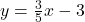Step-by-step explanation:

1) Lines that are parallel to each other have the same slope. The line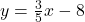is in slope intercept form, or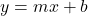form, and the number in place of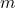represents the slope. Knowing this, it looks likeis in the place of thein that equation, sois the slope – and the slope we need to use for the answer, too.

2) Once you know a point that the line must pass through and a slope, you can write an equation with point-slope form, or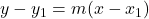.is the slope and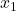and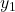are the x and y values of the point it must pass through. So, substitutefor, 0 for, and -3 for. Simplify and isolate y to put it in slope-intercept form: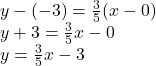3) Thus,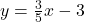is the answer. It’s written in slope-intercept form.